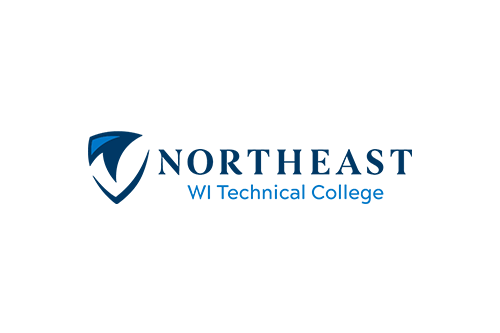# College Algebra & Trig w Apps

Course Number: 10804197
Credits: 5.00

## Course Description

10-804-197 COLLEGE ALGEBRA & TRIGONOMETRY WITH APPLICATIONS …those skills needed for success in Calculus and many application areas on a baccalaureate level. Topics include the real and complex number systems, polynomials, exponents, radicals, solving equations and inequalities (linear and nonlinear), relations and functions, systems of equations and inequalities (linear and nonlinear), matrices, graphing, conic sections, sequences and series, combinatories, and the binomial theorem. (Prerequisite: Next Gen AAF score greater/equal to 250 AND Rdg greater/equal to 250; OR TABE A 9/10 Math greater/equal to no equiv AND Rdg greater/equal to 8.8; OR TABE 11/12 Math greater/equal to no equiv AND Rdg greater/equal to 573; OR ACT Math score greater/equal to 24 AND ACT Rdg greater/equal to 16; OR C or better in Inter Alg-contact academic advisor 920-498-5444)

• Fall
• Spring

## Course Competencies

1. Analyze the features of a graph of a given function or relation
2. Solve linear and quadratic equations and inequalities
3. Analyze the properties of linear and quadratic functions
4. Analyze polynomials
5. Analyze rational functions
7. Analyze exponential and logarithmic functions
8. Solve algebraic systems
9. Analyze conic sections
10. Analyze trigonometric functions
11. Evaluate the trigonometric functions
12. Apply the unit circle and radian measure
13. Analyze graphs of trigonometric functions
14. Analyze inverse trigonometric functions
15. Manipulate trigonometric identities
16. Solve trigonometric equations
17. Solve oblique triangles
18. Complete vector operations
19. Complete operations with complex numbers in trigonometric form
20. Analyze the polar coordinate system